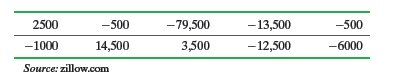×
Get Full Access to Statistics: Informed Decisions Using Data - 5 Edition - Chapter 15.3 - Problem 10
Get Full Access to Statistics: Informed Decisions Using Data - 5 Edition - Chapter 15.3 - Problem 10

×

# ?Housing Prices on Zillow Zillow.com allows people to research real estate listings. One feature is the “Zestimate,” which represents the price ZillowISBN: 9780134133539 240

## Solution for problem 10 Chapter 15.3

Statistics: Informed Decisions Using Data | 5th Edition

• Textbook Solutions
• 2901 Step-by-step solutions solved by professors and subject experts
• Get 24/7 help from StudySoup virtual teaching assistantsStatistics: Informed Decisions Using Data | 5th Edition

4 5 1 235 Reviews
11
0
Problem 10

Housing Prices on Zillow Zillow.com allows people to research real estate listings. One feature is the “Zestimate,” which represents the price Zillow believes the property should sell for. If the Zestimate is accurate, the median difference between the actual selling price and the Zestimate should be zero. The following data represent the difference between the  actual selling price and Zestimate for a random sample of 10 real estate transactions. Do the data suggest the Zestimate is inaccurate? Use the= 0.05 level of significance. Note: A normal probability plot indicates the data are not normal.Step-by-Step Solution:

Step 1 of 5) Housing Prices on Zillow Zillow.com allows people to research real estate listings. One feature is the “Zestimate,” which represents the price Zillow believes the property should sell for. If the Zestimate is accurate, the median difference between the actual selling price and the Zestimate should be zero. The following data represent the difference between the actual selling price and Zestimate for a random sample of 10 real estate transactions. Do the data suggest the Zestimate is inaccurate Use the = 0.05 level of significance. Note: A normal probability plot indicates the data are not normal. However, for skewed distributions, it is often difficult to determine which measure of center is more useful. In some cases, the mean, though not resistant, is easier to find than the median or is more intuitive to the reader within the context of the problem. Here we look at the sampling distributions of the mean and median for two different populations, one normal (symmetric) and one exponential (skewed).

Step 2 of 2

## Discover and learn what students are asking

Statistics: Informed Decisions Using Data : Data Collection
?In Problems 1–5, provide a definition using your own words. 4. Observational study

Statistics: Informed Decisions Using Data : Scatter Diagrams and Correlation
?True or False: If the linear correlation coefficient is close to 0, then the two variables have no relation.

Statistics: Informed Decisions Using Data : Testing the Significance of the Least-Squares Regression Model
?The output shown was obtained from Minitab. (a) The least-squares regression equation is yn = 1.3962x + 12.396. What is the predict

Unlock Textbook Solution